# Introduction to Tree | GATE CSE Notes

Tree is an important topic belonging to the important chapter of Computer Science, i.e. Data Structure. And, when it comes to a competitive examination like GATE, you have to read the whole topic quite deeply. In this article, we have covered all the topics relevant to the tree. We hope the notes for the CSE topics will help you understand this topic in a better way.

## What is a Tree?

A tree is a non-linear and hierarchical data structure that has a group of nodes. When it comes to the tree, each node stores a value.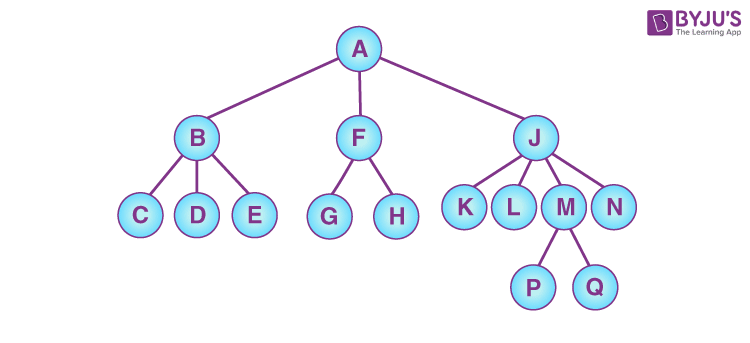## Tree vs No Tree Picture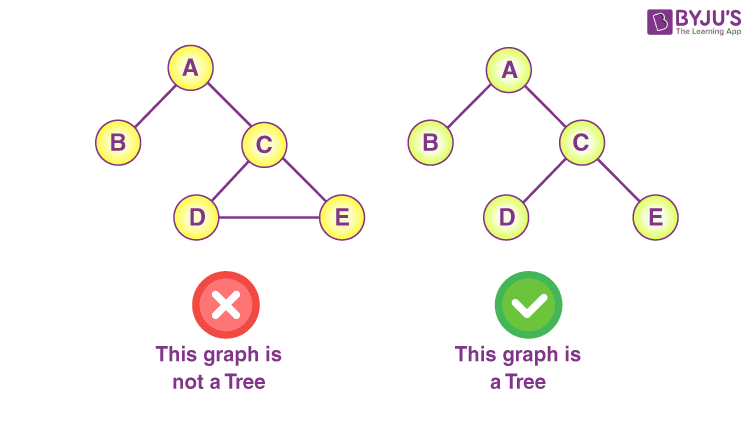## Important Terminologies in Tree

### Node: A node is an entity that contains a key or value and pointers to its child nodes.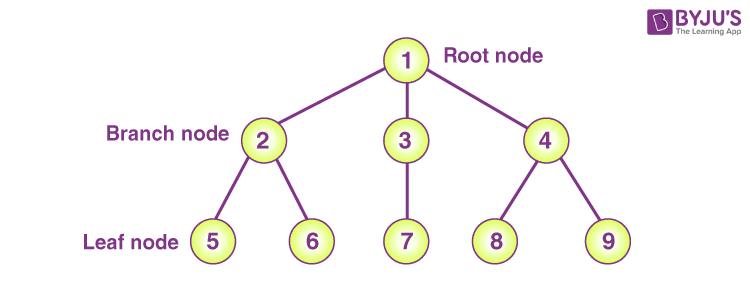### Edge: The connection between any two nodes is known as the edge.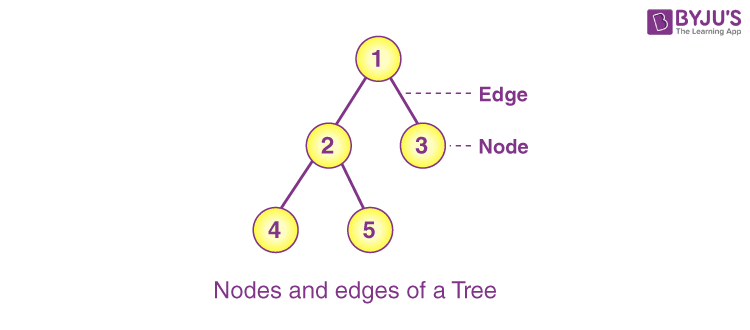### Root: The topmost node of a tree is known as the root.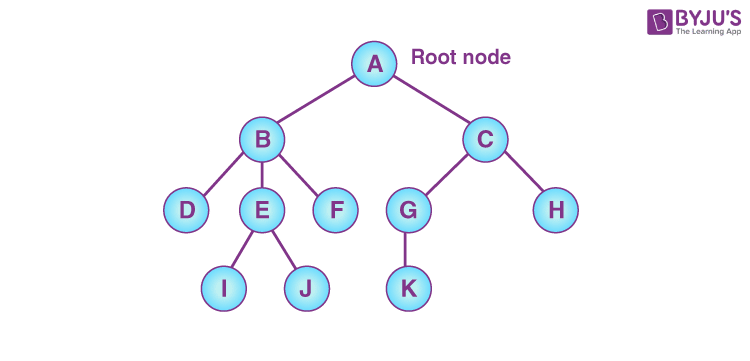### Complete Figure That Explains Tree Terminologies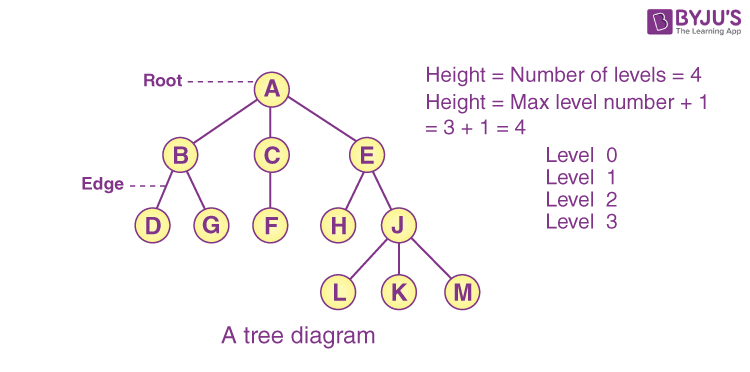## Types of Trees

1. Binary Tree
2. Binary Search Tree
3. AVL Tree
4. B-Tree

## Properties of Trees

• A tree is a hierarchical structure as it contains multiple levels.
• In a tree, the topmost node is known as the root node.
• A node that doesn’t have a child node is known as a leaf node or terminal node.
• The highest number of nodes at every level of i is 2i.
• Height of the tree = the longest path from the root node to the leaf node.
• Depth of a node = the length of the path to its root.

## Tree Applications

• Trees store data in the form of a hierarchical structure.
• The tree is used to manage the data efficiently. It improves the following process like insertion, deletion and searching.
• Tree is also utilised to keep the data in routing tables in the routers.

## Practice Problem – Tree

Q. Let T be a full binary tree with 8 leaves. (A full binary tree has every level full.) Suppose two leaves a and b of T are chosen uniformly and independently at random. The expected value of the distance between a and b in T (i.e., the number of edges in the unique path between a and b) is rounded off to 2 decimal places.

Q. Let T be a binary search tree with 15 nodes. The minimum and maximum possible heights of T are:

Note: The height of a tree with a single node is 0

• (A) 4 and 15, respectively
• (B) 3 and 14, respectively
• (C) 4 and 14, respectively
• (D) 3 and 15, respectively

Q. The postorder traversal of a binary tree is 8,9,6,7,4,5,2,3,1. The inorder traversal of the same tree is 8,6,9,4,7,2,5,1,3. The height of a tree is the length of the longest path from the root to any leaf. The height of the binary tree above is ______.

Keep learning and stay tuned to get the latest updates on GATE Exam along with GATE Eligibility CriteriaGATE 2023GATE Admit CardGATE Syllabus for CSE (Computer Science Engineering)GATE CSE NotesGATE CSE Question Paper, and more.

Also Explore,